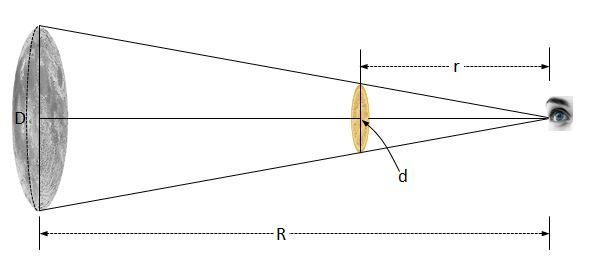# Measuring the diameter of the moonDid you know you can approximate the diameter of the moon with a coin $($of diameter $d)$ placed a distance $r$ in front of your eye?

If the distance between the moon and your eye is $R,$ what is the diameter of the moon?

×